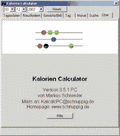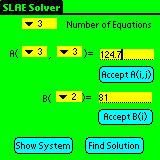## Simple Risk Calculator for PocketPC PDA

 Simple risk calculator calculates a set of risk measures, such as VaR, CVaR, dispersions, and risk\reward ratios. The calculator is useful for decision making, risk engineering, risk measurement, and risk management tasks. Download trial   Buy now (\$14.00) Vendor: AdvMathAppl

Programs similar to Simple Risk Calculator for PocketPC PDA

1. Simple Risk Calculator for Windows
Simple risk calculator calculates a set of risk measures , such as VaR, CVaR, dispersions, and risk\reward ratios. The calculator is useful for decision making, risk engineering, risk measurement, and risk management tasks.
2. Simple Risk Calculator for Palm
3. Forex Arbitrage Calculator for PDA

4. Investment and Mortgage Loan CalculatorLoan Calculator. Lump Sum Calculator. Split Loan Calculator. Equity Loan Calculator. Term Deposit Calculator. Savings Plan Calculator. Loan Comparison Calculator. Extra Repayment Calculator. Borrowing Limit Calculator.
Vendor: Charuka Abhayawickrama

5. BlackJack Calculator for PDA (Palm OS)
BJ_Calculator allows to calculate theoretical advantage of playing in Blackjack under given conditions with a fixed strategy.
Platform: Palm OS

6. Kalorien Calculator für PCCalculates weight, kalories, joule, etc.
Platform: Windows NT, Windows 2000, Windows XP, Windows 98, Windows ME
Language: English, German
Vendor: Markus Schneider
7. Kalorien Calculator

8. SLAE Solver for PocketPCSLAE SOLVER allows to find on a PDA high accuracy solutions of linear algebraic systems with N equations.